# 1 Introduction

创新点：利用图灰盒攻击进行对比学习。

# 2 Graph robust contrastive learning

## 2.1 Background

目的：期望同一节点在两个视图的嵌入是相似的，不同节点的嵌入在两个图视图之间的嵌入是不同的。如下图所示：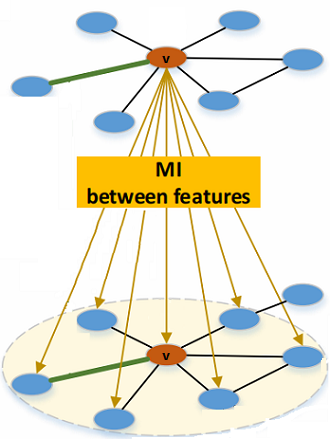通过下式计算编码器的参数 $\theta$ ：

$\underset{\theta}{ \arg \max } \;\;\; \mathbb{E}_{\tau_{1}, \tau_{2} \sim \mathrm{T}}\left[\sum\limits _{v \in V} \sigma\left(z_{1}, z_{2}\right)-\sum\limits_{u \in N e g(v)} \sigma\left(z_{1}, f_{\theta}(u)\right)\right]$

其中

• $z_{1} \equiv f_{\theta}\left(\tau_{1}(v)\right)$ ， $z_{2} \equiv f_{\theta}\left(\tau_{2}(v)\right)$；
• $N e g(v)=\left\{\tau_{1}(u) \mid u \in V \backslash\{v\}\right\} \cup\left\{\tau_{2}(u) \mid u \in V \backslash\{v\}\right\}$ 是两个图视图中除 $v$ 以外的节点；
• $\sigma$ 是相似性度量 Sigmoid 函数；

由于数据增强 $T$  的搜索空间较大，上述优化问题难以解决。采用 GRACE 方法来解决这个问题。

即：先对 $z_{1}, z_{2}$ 施加一个两层的 MLP ，然后再计算 Sigmoid ，损失函数变为：【GRACE 是计算余弦相似度】

$\frac{1}{2 n} \sum\limits _{v \in V}\left[\mathcal{L}\left(v, \tau_{1}, \tau_{2}\right)+\mathcal{L}\left(v, \tau_{2}, \tau_{1}\right)\right]\quad\quad\quad(1)$

其中：

$\mathcal{L}\left(v, \tau_{1}, \tau_{2}\right)=-\log {\Large \frac{\exp \left(\sigma\left(z_{1}, z_{2}\right) / t\right)}{\exp \left(\sigma\left(z_{1}, z_{2}\right) / t\right)+\sum\limits _{u \in N e g(v)} \exp \left(\sigma\left(z_{1}, f_{\theta}(u)\right) / t\right)}}$

## 2.2 Motivation

从白盒攻击之基于积分梯度的攻击的角度考虑问题：白盒攻击表示攻击者可以获得被攻击模型的完整信息，所有的信息都是已知的

## 2.3 Method

GROC算法如图 1 所示，详见算法1。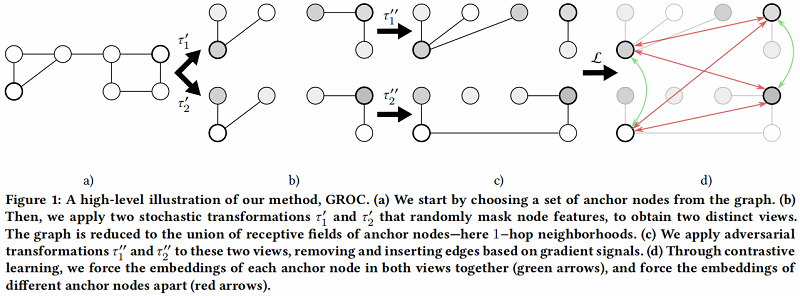图解如下：

• 从原图中选择一个锚点集合 $B_i$（大小 $b=2$），在该图描点集合包括 $2$ 个节点；
• 对锚点集合及其 $\text{1-hop}$ 分别以隐藏率 $p_1,p_2$ 进行特征隐藏 $\tau_{1}^{\prime}$、 $\tau_{2}^{\prime}$ ，并计算两个视图对应的 $S^+$、$S^-$；
• 对两个视图进行对抗性转换 $\tau_{1}^{\prime \prime}$  和 $\tau_{2}^{\prime \prime}$ ；【先将 $S^+$ 以权重 $1/|S^{+}|$ 暂时添加到图中，走一次网络，计算 $\text{Eq.1}$ 损失（仅考虑 $B_i$ 中的节点），从而可以计算出边上的梯度 $S_{i j}=\frac{\partial l(v)}{\partial A_{i j}}$ ，再从 $S^{-}$ 中删除 $q_{i}^{-} \cdot\left|S^{-}\right|$ 条梯度最小的边，从 $S^{+}$ 添加 $q_{i}^{+} \cdot\left|S^{+}\right|$ 条梯度最大的边 】；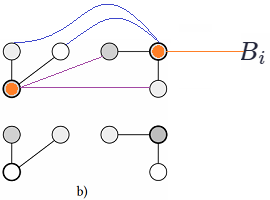• 走一次网络，并计算$B_i$ 中的节点损失 $\text{Eq.1}$；

算法如下：灰盒攻击，攻击者不能得到被攻击模型的结构和参数，只能得到训练的数据。灰盒攻击通常不是直接攻击给定的模型，而是首先利用训练数据训练一个代理模型，然后攻击这个代理模型。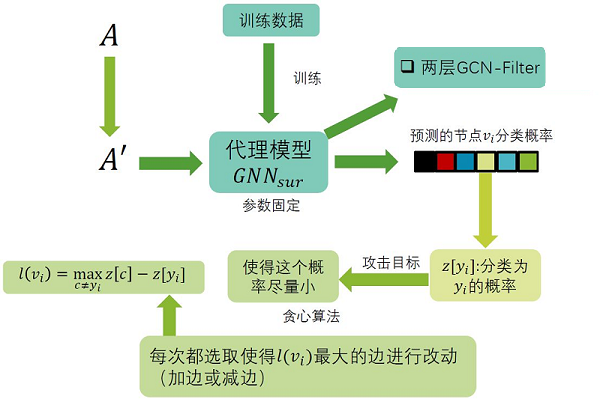# 3 Experiments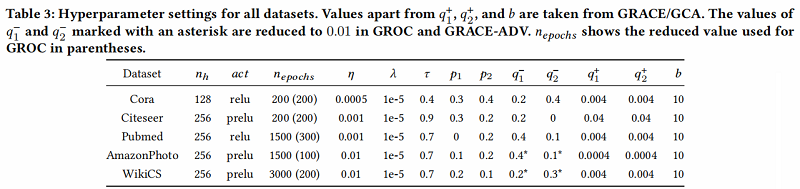参数：

• 编码器 $f_{\theta}$ 是两层的 GCN ，每层的大小分别为：$2n_h$ 、$n_h$
• 学习率：$\eta$
• 训练次数：$n_{epoch}$
• L2惩罚项的惩罚因子：$\lambda$
• 温度参数：$\tau$
• 特征掩蔽率：$p_1$、$p_2$
• 边删除率：$q_{1}^{-}$、$q_{2}^{-}$
• 边插入率：$q_{1}^{+}$、$q_{2}^{+}$
• 节点批次大小 ：$b$# 4 Conclusion

在本研究中，我们关注图形自监督学习方法的对抗鲁棒性问题。我们怀疑，并且通过后来的实验证实，先前引入的对比学习方法很容易受到对抗性攻击。作为在这种情况下实现鲁棒性的第一步，我们引入了一种新的方法，GROC，它通过引入对抗性转换和边缘插入来增强图视图的生成。我们通过一组初步的实验证实了该方法可以提高所产生的表示的对抗性鲁棒性。我们希望这项工作最终将导致在图上产生更成功和更鲁棒的对比学习算法。

# 相关论文微小扰动对高精度 GNNs 任然有影响：[7, 25, 31]

posted @ 2022-04-24 22:10  关注我更新论文解读  阅读(189)  评论(0编辑  收藏  举报